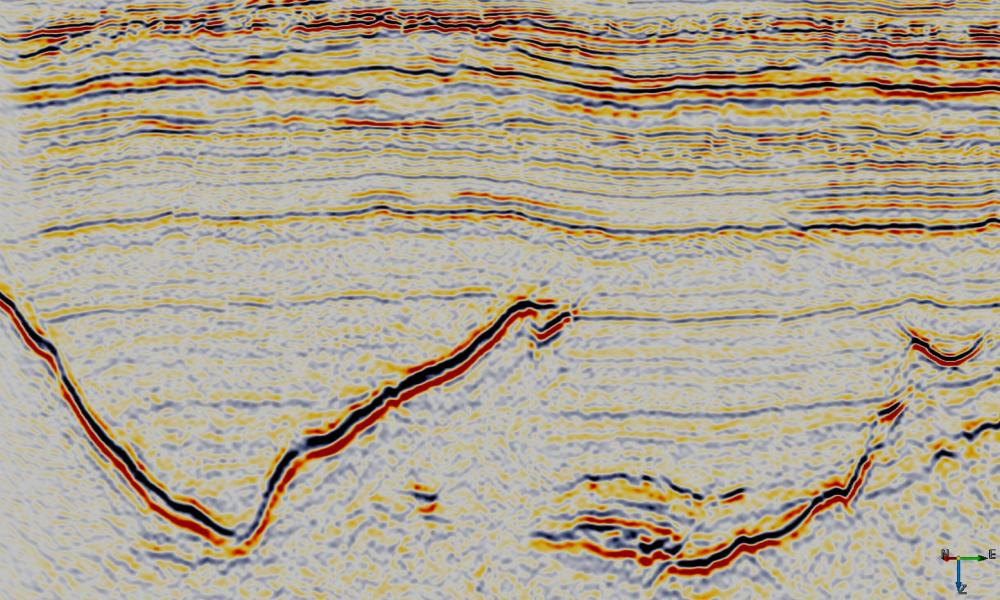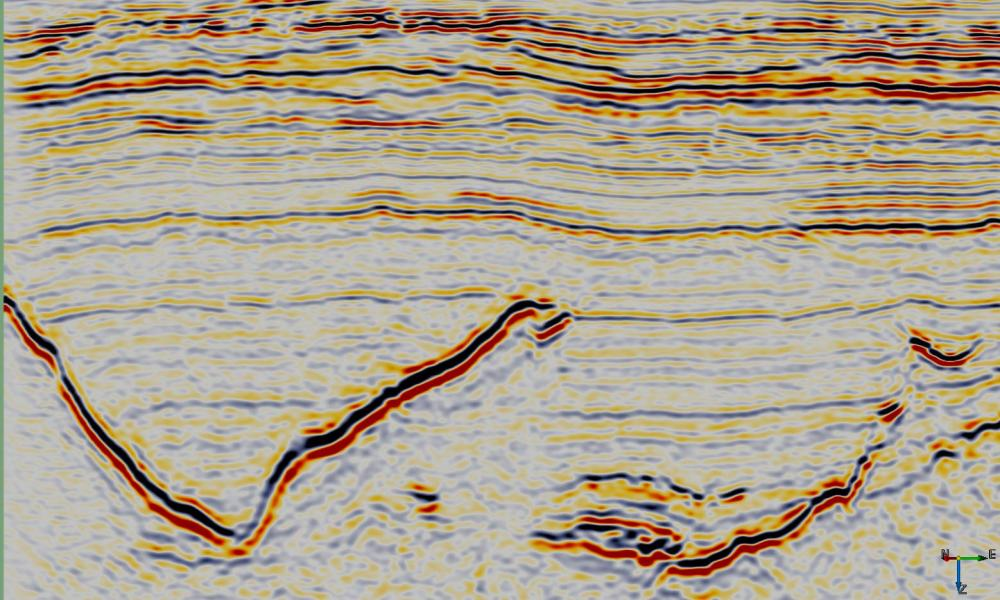Script: ex_lpa_smooth.py

### Description

This Python ExternalAttrib script can be used to filter noise while preserving steep dips. A region of data around each sample location is approximated by a second order 3D polynomial using gaussian weighted least squares.

The approximation has the following form:

$$r_0+ r_1 * x + r_2 * y + r_3 * z + r_4 * x^2 + r_5 * y^2 + r_6 * z^2 +r_7 * x * y + r_8 * x * z + r_9 * y * z$$

where x (inline), y (crossline) and z (time/depth) are relative to the analysis location, ie the analysis location has x=y=z=0.

This attribute calculates and outputs only the $r_0$ term of the local polynomial approximation. This provides a smoother version of the input with relatively minor smearing of steep dips and fault cuts. Increasing either the Weight Factor or size of the analysis volume (StepOut or Z window) increases the amount of smoothing.

### Examples### Input Parameters

Z window (+/-samples) Specifies the extent of the analysis cube in the Z direction. Number of Z samples in cube will be $2 * Zwindow + 1$.
Stepout Specifies the inline and crossline extent of the analysis cube. Number of samples in each direction will be $2 * Stepout + 1$.
Weight Factor Determines the extent of the gaussian weight function used in the weighted least squares approximation. The standard deviation of the gaussian weight function ($\sigma$) is related to this value by $$\sigma = min(2*Stepout, 2*Zwindow) * WeightFactor$$ A value of 0.15 gives near zero weight for points at the smallest extent of the analysis cube.